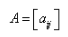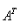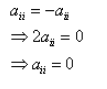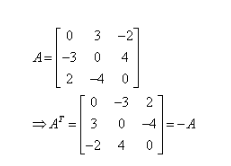# Define skew-symmetric matrix with the help of an example

Define skew-symmetric matrix with the help of an example.

A square matrixis said to be skew symmetric matrix if= -A, that isfor all possible values of i and j. Now, if we put i = j, we
haveThis means that all the diagonal elements of a skew symmetric matrix are zero.
For example: